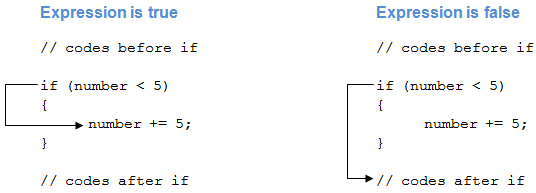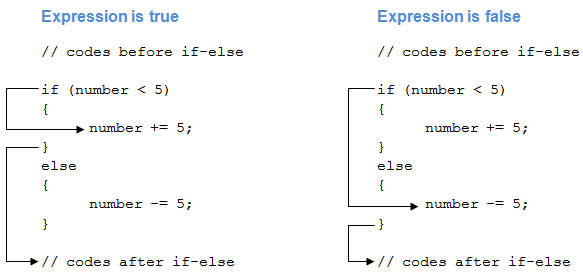C# if, if...else, if...else if and Nested if Statement

# C# if, if...else, if...else if and Nested if Statement

#### In this article, we will learn how to use if, if...else, if...else if statement in C# to control the flow of our program’s execution.

Testing a condition is inevitable in programming. We will often face situations where we need to test conditions (whether it is `true` or `false`) to control the flow of program. These conditions may be affected by user's input, time factor, current environment where the program is running, etc.

In this article, we'll learn to test conditions using if statement in C#.

## C# if (if-then) Statement

C# if-then statement will execute a block of code if the given condition is true. The syntax of if-then statement in C# is:

```if (boolean-expression)
{
// statements executed if boolean-expression is true
}
```
• The boolean-expression will return either true or `false`.
• If the boolean-expression returns `true`, the statements inside the body of if ( inside `{...}` ) will be executed.
• If the boolean-expression returns `false`, the statements inside the body of if will be ignored.

For example,

```if (number < 5)
{
number += 5;
}
```

In this example, the statement

`number += 5;`

will be executed only if the value of number is less than 5.

Remember the += operator?

### How if statement works?### Example 1: C# if Statement

``````using System;

namespace Conditional
{
class IfStatement
{
public static void Main(string[] args)
{
int number = 2;
if (number < 5)
{
Console.WriteLine("{0} is less than 5", number);
}

Console.WriteLine("This statement is always executed.");
}
}
}
``````

When we run the program, the output will be:

```2 is less than 5
This statement is always executed.```

The value of number is initialized to 2. So the expression `number < 5` is evaluated to `true`. Hence, the code inside the if block are executed. The code after the if statement will always be executed irrespective to the expression.

Now, change the value of number to something greater than `5`, say `10`. When we run the program the output will be:

`This statement is always executed.`

The expression `number < 5` will return `false`, hence the code inside if block won't be executed.

## C# if...else (if-then-else) Statement

The if statement in C# may have an optional else statement. The block of code inside the else statement will be executed if the expression is evaluated to `false`.

The syntax of if...else statement in C# is:

```if (boolean-expression)
{
// statements executed if boolean-expression is true
}
else
{
// statements executed if boolean-expression is false
}
```

For example,

```if (number < 5)
{
number += 5;
}
else
{
number -= 5;
}
```

In this example, the statement

`number += 5;`

will be executed only if the value of number is less than `5`.

The statement

`number -= 5;`

will be executed if the value of number is greater than or equal to `5`.

### How if...else Statement works?### Example 2: C# if...else Statement

``````using System;

namespace Conditional
{
class IfElseStatement
{
public static void Main(string[] args)
{
int number = 12;

if (number < 5)
{
Console.WriteLine("{0} is less than 5", number);
}
else
{
Console.WriteLine("{0} is greater than or equal to 5", number);
}

Console.WriteLine("This statement is always executed.");
}
}
}
``````

When we run the program, the output will be:

```12 is greater than or equal to 5
This statement is always executed.```

Here, the value of number is initialized to `12`. So the expression `number < 5` is evaluated to `false`. Hence, the code inside the else block are executed. The code after the if..else statement will always be executed irrespective to the expression.

Now, change the value of number to something less than `5`, say `2`. When we run the program the output will be:

```2 is less than 5
This statement is always executed.```

The expression `number < 5` will return true, hence the code inside if block will be executed.

Ternary operator in C# provides a shortcut for C# if...else statement.

## C# if...else if (if-then-else if) Statement

When we have only one condition to test, if-then and if-then-else statement works fine. But what if we have a multiple condition to test and execute one of the many block of code.

For such case, we can use if..else if statement in C#. The syntax for if...else if statement is:

```if (boolean-expression-1)
{
// statements executed if boolean-expression-1 is true
}
else if (boolean-expression-2)
{
// statements executed if boolean-expression-2 is true
}
else if (boolean-expression-3)
{
// statements executed if boolean-expression-3 is true
}
.
.
.
else
{
// statements executed if all above expressions are false
}
```

The if...else if statement is executed from the top to bottom. As soon as a test expression is `true`, the code inside of that if ( or else if ) block is executed. Then the control jumps out of the if...else if block.

If none of the expression is `true`, the code inside the else block is executed.

Alternatively, we can use switch statement in such condition.

### Example 3: C# if...else if Statement

``````using System;

namespace Conditional
{
class IfElseIfStatement
{
public static void Main(string[] args)
{
int number = 12;

if (number < 5)
{
Console.WriteLine("{0} is less than 5", number);
}
else if (number > 5)
{
Console.WriteLine("{0} is greater than 5", number);
}
else
{
Console.WriteLine("{0} is equal to 5");
}
}
}
}
``````

When we run the program, the output will be:

`12 is greater than 5`

The value of number is initialized to `12`. The first test expression `number < 5` is `false`, so the control will move to the else if block. The test expression `number > 5` is `true` hence the block of code inside else if will be executed.

Similarly, we can change the value of `number` to alter the flow of execution.

## Nested if...else Statement

An if...else statement can exist within another if...else statement. Such statements are called nested if...else statement.

The general structure of nested if…else statement is:

```if (boolean-expression)
{
if (nested-expression-1)
{
// code to be executed
}
else
{
// code to be executed
}
}
else
{
if (nested-expression-2)
{
// code to be executed
}
else
{
// code to be executed
}
}
```

Nested if statements are generally used when we have to test one condition followed by another. In a nested if statement, if the outer if statement returns true, it enters the body to check the inner if statement.

### Example 4: Nested if...else Statement

The following program computes the largest number among 3 numbers using nested if...else statement.

``````using System;

namespace Conditional
{
class Nested
{
public static void Main(string[] args)
{
int first = 7, second = -23, third = 13;
if (first > second)
{
if (firstNumber > third)
{
Console.WriteLine("{0} is the largest", first);
}
else
{
Console.WriteLine("{0} is the largest", third);
}
}
else
{
if (second > third)
{
Console.WriteLine("{0} is the largest", second);
}
else
{
Console.WriteLine("{0} is the largest", third);
}
}
}
}
}
``````

When we run the program, the output will be:

`13 is the largest`